2015/09/08 21:48

# 编辑文本及键盘输入

InputScope inputScope = new InputScope();
InputScopeName inputScopeName= new InputScopeName();
inputScopeName.NameValue = InputScopeNameValue.TelephoneNumber;
phoneNumberTtBox.InputScope = scope;

phoneNumberTtBox.InputScope = new InputScope()
{
Names = {new InputScopeName(InputScopeNameValue.TelephoneNumber)}
};


# 情节提要动画与关键帧动画

## 简单动画示例

<Grid Background="{ThemeResource ApplicationPageBackgroundThemeBrush}">
<ItemsControl Grid.Row="1" x:Name="itemsControlRectangle">
<ItemsControl.ItemContainerTransitions>
<TransitionCollection>
<EntranceThemeTransition/>
</TransitionCollection>
</ItemsControl.ItemContainerTransitions>
<ItemsControl.ItemsPanel>
<ItemsPanelTemplate>
<WrapGrid Height="400"/>
</ItemsPanelTemplate>
</ItemsControl.ItemsPanel>

<ItemsControl.Items>
<Rectangle Fill="Red" Width="100" Height="100" Margin="12"/>
<Rectangle Fill="Wheat" Width="100" Height="100" Margin="12"/>
<Rectangle Fill="Yellow" Width="100" Height="100" Margin="12"/>
<Rectangle Fill="Blue" Width="100" Height="100" Margin="12"/>
<Rectangle Fill="Green" Width="100" Height="100" Margin="12"/>
<Rectangle Fill="Gray" Width="100" Height="100" Margin="12"/>
<Rectangle Fill="White" Width="100" Height="100" Margin="12"/>
<Rectangle Fill="Gainsboro" Width="100" Height="100" Margin="12"/>
<Rectangle Fill="Magenta" Width="100" Height="100" Margin="12"/>
<Rectangle Fill="NavajoWhite" Width="100" Height="100" Margin="12"/>
<Rectangle Fill="Khaki" Width="100" Height="100" Margin="12"/>
</ItemsControl.Items>
</ItemsControl>
</Grid>

## 情节提要动画

<Page.Resources>
<Storyboard x:Name="storyboardRectangle" >
<DoubleAnimation  Storyboard.TargetName="rectangle" Storyboard.TargetProperty="Opacity" From="1.0" To="0" Duration="0:0:1" AutoReverse="True" RepeatBehavior="Forever"/>
</Storyboard>
</Page.Resources>

<Grid>
<Rectangle x:Name="rectangle" Width="200" Height="130" Fill="Blue"/>
</Grid>

storyboardRectangle.Begin();

Storyboard.TargetProperty="(rectangle.Fill).(SolidColorBrush.Color)"

(UIElement.RenderTransform).(TranslateTransform.X) 应用到RenderTransform上，并创建TranslateTransform的X值的动画

(UIElement.RenderTransform).(TransformGroup.Children).(TranslateTransform.X) 应用到RenderTransform上，并创建TranslateTransform

## 关键帧动画

1.线性关键帧

2.样条关键帧

3.缓动关键帧

BackEase：动画开始在指定路径上运动前稍微收缩动画的运行。
BounceEase：创建回弹效果。
CircleEase：使用圆函数创建加速或减速的动画。
CubicEase：使用函数 f(t) = t3 创建加速或减速的动画。
ElasticEase：创建一个动画，模拟弹簧的来回振荡运动，直到它达到停止状态。
ExponentialEase：使用指数公式创建加速或减速的动画。
PowerEase：使用公式 f(t) = tp 创建加速或减速的动画，其中 p 等于 Power 属性。
QuarticEase：使用函数 f(t) = t4 创建加速或减速的动画。
QuinticEase：使用函数 f(t) = t5 创建加速或减速的动画。
SineEase：使用正弦公式创建加速或减速的动画。

# 绘制图形

## Rectangle

<Rectangle Fill="Yellow" Width="300" Height="200" Stroke="Blue" StrokeThickness="10" RadiusX="80" RadiusY="40"/>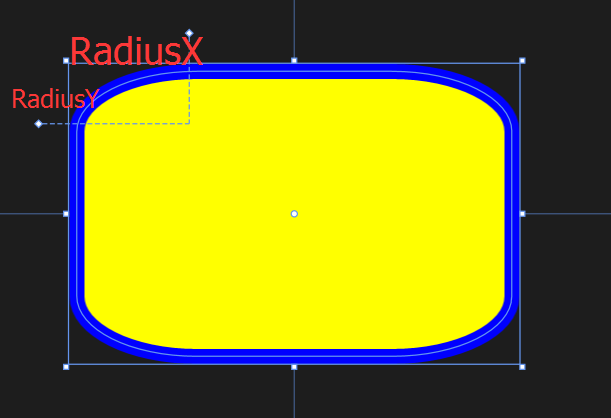## Ellipse

<Ellipse Fill="Blue" Height="200" Width="350"/>

## Polygon

Polygon则显得比较自由，只需要定义出各个顶点，它就会将这些点连接起来。那么我们可能会有疑问，需不需要确定图形的起始点和终点呢？答案是不用的，因为Polygon会自动将终点和起始点连接起来（它会假设图形是闭合的）。

<Polygon Fill="Green" Points="0,0,100,0,100,100,0,100 "/>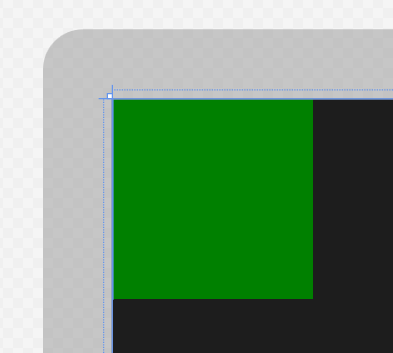## Line

Line的使用也比较简单，但有一点要注意，必须设置好Stroke和StrokeThickness的属性值，否则Line就不会显示出来。原因很简单，因为它是直线。

<Line Stroke="Red" StrokeThickness="10" X1="100" Y1="0" Y2="400" X2="400"/>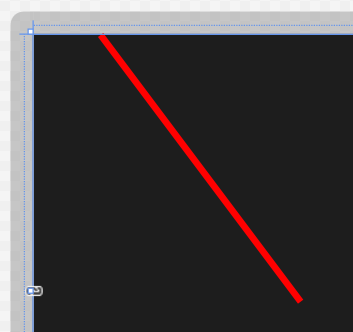## Path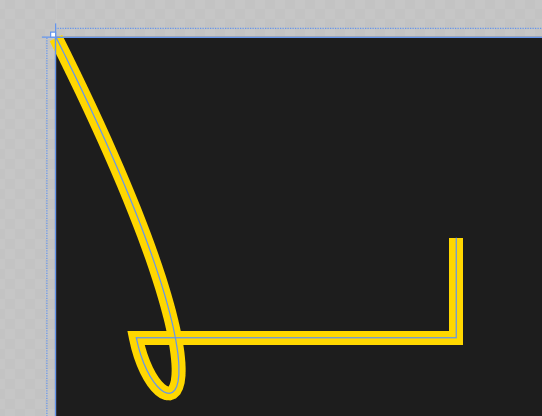<Path Stroke="Gold" StrokeThickness="7" Data="M 0,0 C 100,200 50,200 40,150 H 200 V 100 "/>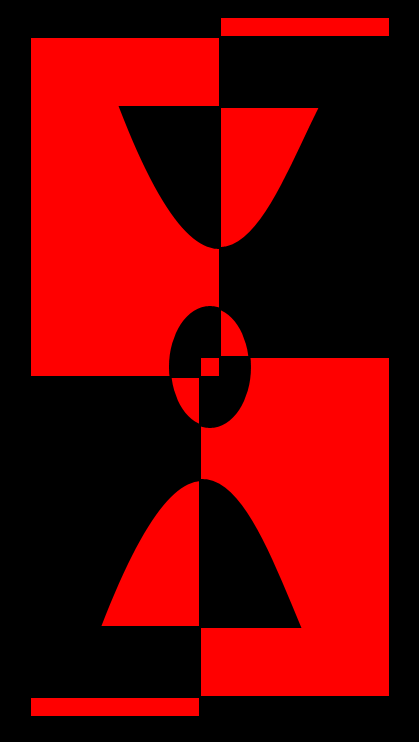<Path Stroke="Black" StrokeThickness="1" Fill="red">
<Path.Data>
<GeometryGroup>
<RectangleGeometry Rect="5,5 180,10" />
<RectangleGeometry Rect="5,5 95,180" />
<RectangleGeometry Rect="90,175 95,180"/>
<RectangleGeometry Rect="5,345 180,10" />
<PathGeometry>
<PathGeometry.Figures>
<PathFigureCollection>
<PathFigure IsClosed="true" StartPoint="50,50">
<PathFigure.Segments>
<PathSegmentCollection>
<BezierSegment Point1="100,180" Point2="125,100" Point3="150,50"/>
</PathSegmentCollection>
</PathFigure.Segments>
</PathFigure>
<PathFigure IsClosed="true" StartPoint="40,310">
<PathFigure.Segments>
<PathSegmentCollection>
<BezierSegment Point1="90,180" Point2="115,250" Point3="140,310"/>
</PathSegmentCollection>
</PathFigure.Segments>
</PathFigure>
</PathFigureCollection>
</PathGeometry.Figures>
</PathGeometry>
</GeometryGroup>
</Path.Data>
</Path>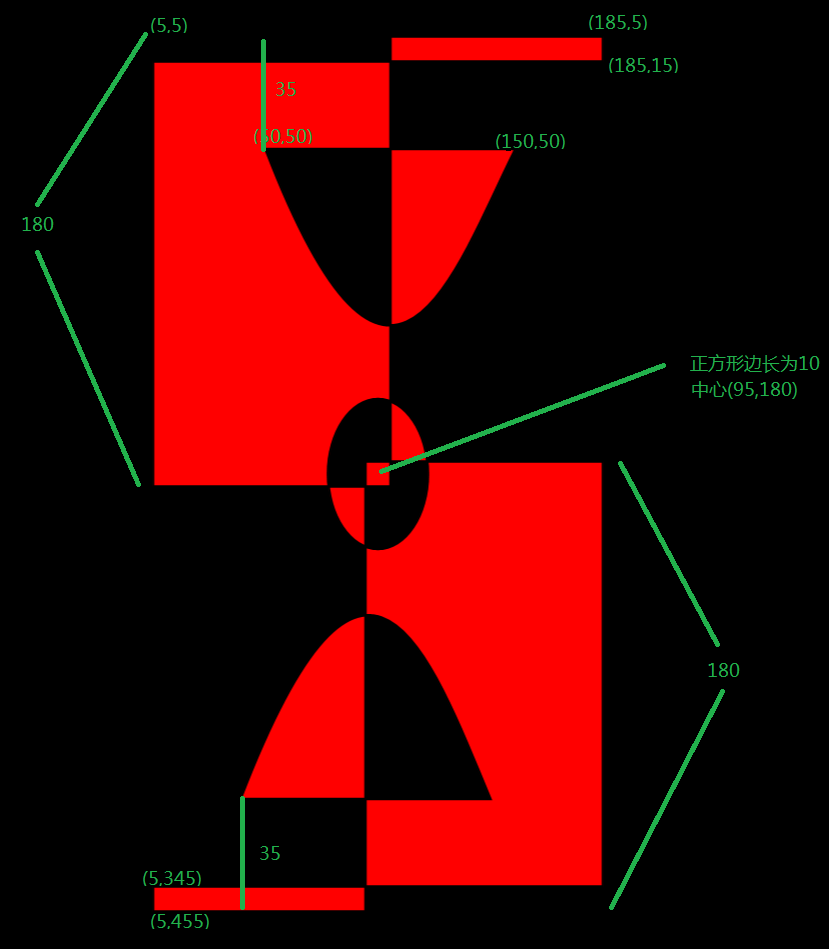# 画笔与图像

## 纯色画笔

<Rectangle Width="200" Height="100" Fill="#FF550088" />

  <Rectangle Width="200" Height="100">
<Rectangle.Fill>
<SolidColorBrush Color="Yellow" Opacity="0.3" />
</Rectangle.Fill>
</Rectangle>

## 渐变画笔

   <Rectangle Width="200" Height="100">
<Rectangle.Fill>
</Rectangle.Fill>
</Rectangle>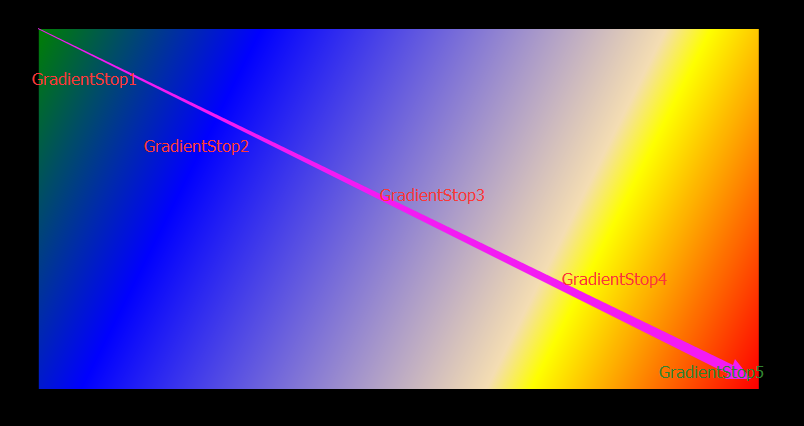## 直接添加图片

 <Ellipse Height="100" Width="200">
<Ellipse.Fill>
<ImageBrush ImageSource="9327.jpg"/>
</Ellipse.Fill>
</Ellipse>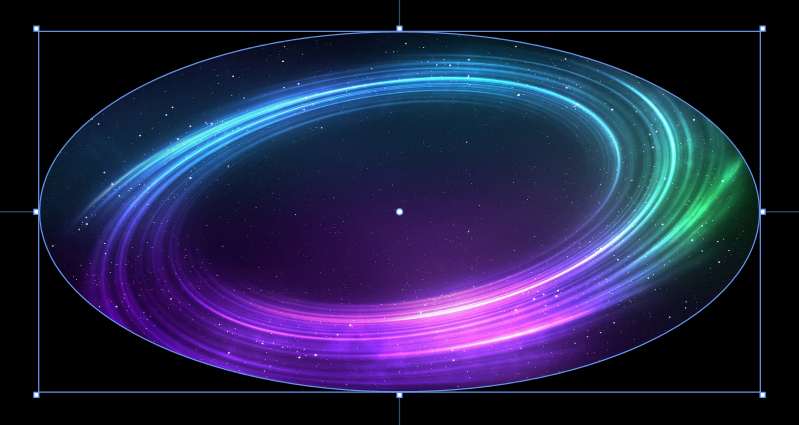## Stretch属性

None：图像不经过拉伸。如果源图像比所留给Image的区域大，那么就会被剪切。
Uniform：按照纵横比来缩放图像。
UniformToFill：按照纵横比来填满所有区域，这意味着可能会有一部分不可见。
Fill。因为不保留纵横比而填满屏幕，所以图像部分全部可见，但会产生画面变形（失真）。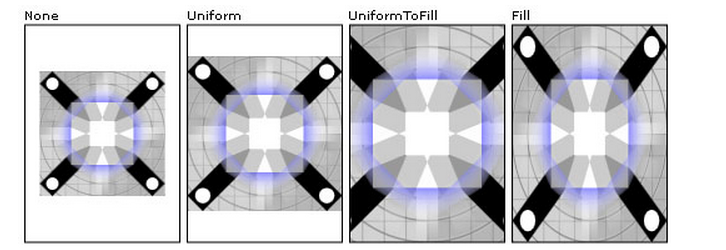## Clip属性

<Image Source="9327.jpg">
<Image.Clip>
<RectangleGeometry Rect="10,10,100,100"/>
</Image.Clip>
</Image>

Image和ImageBrush能处理的图像格式有如下几种：

JPEG XR

0
0 收藏

### 作者的其它热门文章0 评论
0 收藏
0